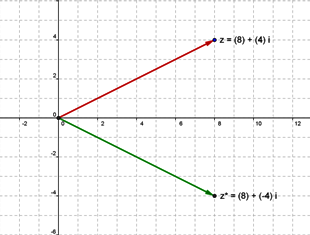The complex conjugate of a complex number $z$ is denoted $z^*$ or $\bar{z}.$

If $z=x+iy$ then

• $z^*=\bar{z}=x-iy$
The complex conjugate $z^*$ is the mirror image of $z$ in the real line.